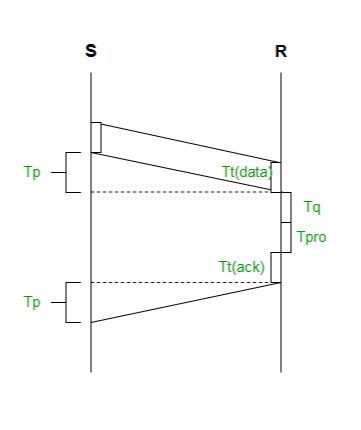# Efficiency of Stop and Wait Protocol

Stop and Wait is a flow control protocol. In which the sender sends one packet and waits for the receiver to acknowledge and then it will send the next packet. In case if the acknowledgment is not received, the sender will retransmit the packet. This is the simplest one and easy to implement. but the main disadvantage is the efficiency is very low.Total time taken to send one packet,

```= Tt(data) + Tp(data) + Tq + Tpro + Tt(ack) + Tp(ack)

Since,
Tp(ack) = Tp(data)

And,
Tt(ack) << Tt(data).

So we can neglect Tt(ack)
Tq = 0 and Tpro = 0

Hence,
Total time = Tt(data) + 2 * Tp ```

Where,

```Tt(data) : Transmission delay for Data packet
Tp(data) : propagation delay for Data packet
Tq: Queuing delay
Tpro: Processing delay
Tt(ack): Transmission delay for acknowledgment
Tp(ack) : Propagation delay for acknowledgment ```

We know that the Efficiency (η),

```= Useful time / Total cycle time.
= Tt / (Tt + 2*Tp)
= 1 / (1+2*(Tp/Tt))
= 1 / (1+2*a)

where,
a = Tp / Tt ```

Throughput: Number of bits send per second, which is also known as Effective Bandwidth or Bandwidth utilization.

```Throughput,
= L/(Tt + 2*Tp)
= ((L/BW)*BW)/(Tt + 2*Tp)
= Tt/(Tt + 2*Tp) * BW
= 1/(1 + 2a) * BW

Hence, Throughput
= η * BW

where,
BW : BandWidth
L : Size of Data packet ```

Factors affecting Efficiency:

```n = 1/(1 + 2*(Tp/Tt)
= 1/(1 + 2*(d/v)*(BW/L))

where,
d = distance between source and receiver
v = velocity ```

Lets see an example.

Example:
Given,

```Tt = 1ms
Tp = 2ms
Bandwidth = 6 Mbps

Efficiency(η)
= 1/(1 + 2*a)
= 1/(1 + 2*(2/1))
= 1/5
= 20 %

Throughput
= η * BW
= (1/5) * 6
= 1.2 Mbps ```

Note:
As we can observe from the above given formula of Efficiency that:

1. On increasing the distance between source and receiver the Efficiency will decrease. Hence, Stop and Wait is only suitable for small area network like LAN. It is not suitable for MAN or WAN, as the efficiency will be very low.
2. If we increase the size of the Data packet, the efficiency is going to increase. Hence, it is suitable not for small packets. Big data packets can be send by Stop and Wait efficiently.My Personal Notes arrow_drop_upCheck out this Author's contributed articles.

If you like GeeksforGeeks and would like to contribute, you can also write an article using contribute.geeksforgeeks.org or mail your article to contribute@geeksforgeeks.org. See your article appearing on the GeeksforGeeks main page and help other Geeks.

Please Improve this article if you find anything incorrect by clicking on the "Improve Article" button below.

Improved By : arifhbd120

Article Tags :
Practice Tags :

2

Please write to us at contribute@geeksforgeeks.org to report any issue with the above content.